# How to Draw Four Dimensional Figures

• Author:
• Updated date:

I am an online writer who enjoys writing about mathematics and science.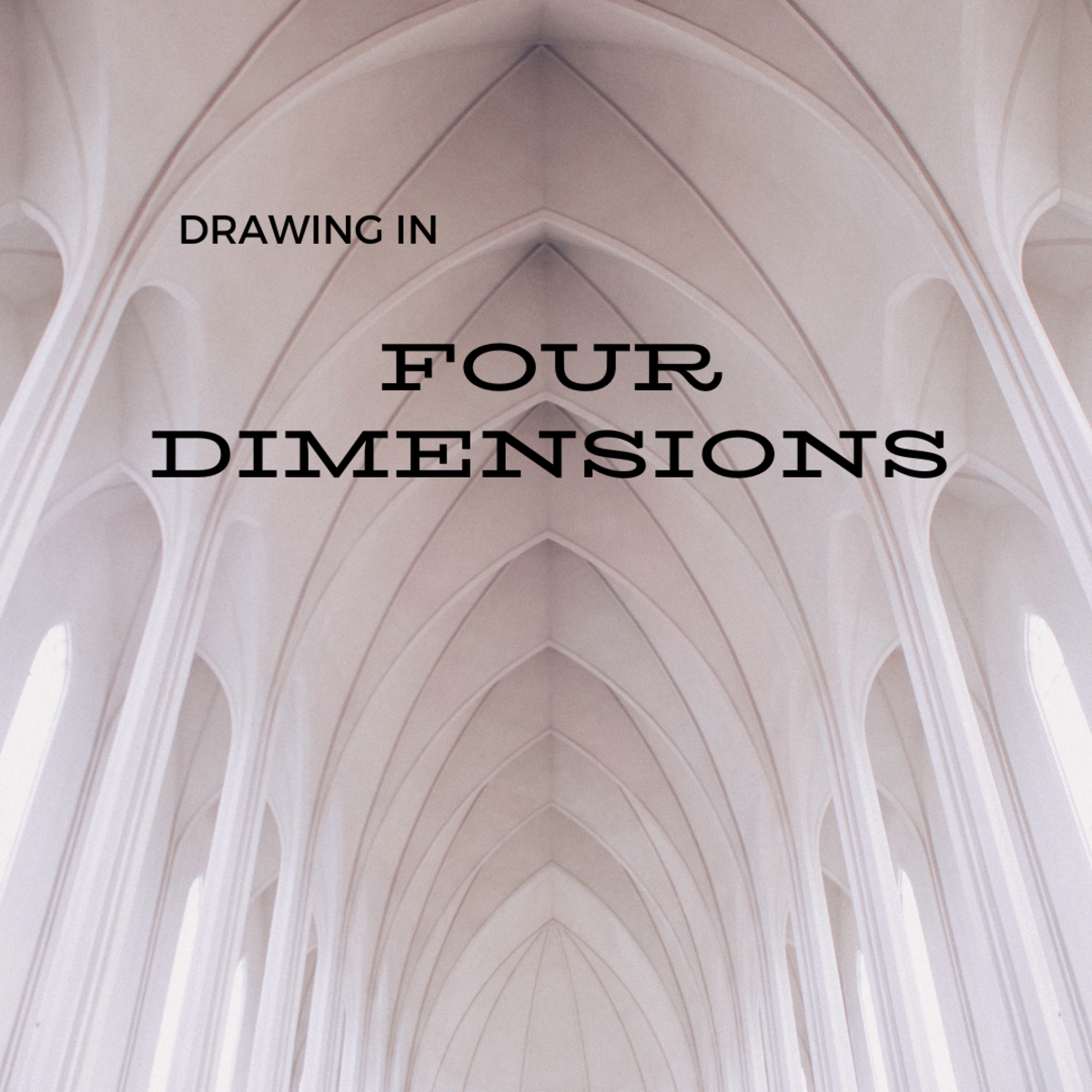In this article we will see how to draw a two-dimensional representation of a four-dimensional object.Canva

## Let's Begin with the Basics

Fig. 1 shows the x-axis or number line. This is a single dimension. Any point on the line is represented by a single number (+x or -x) that indicates its distance from the origin (0).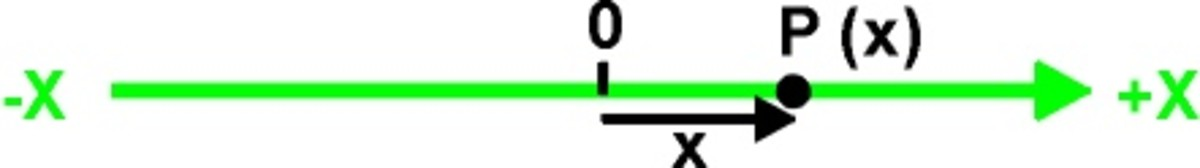Fig. 1. The number line showing 1D space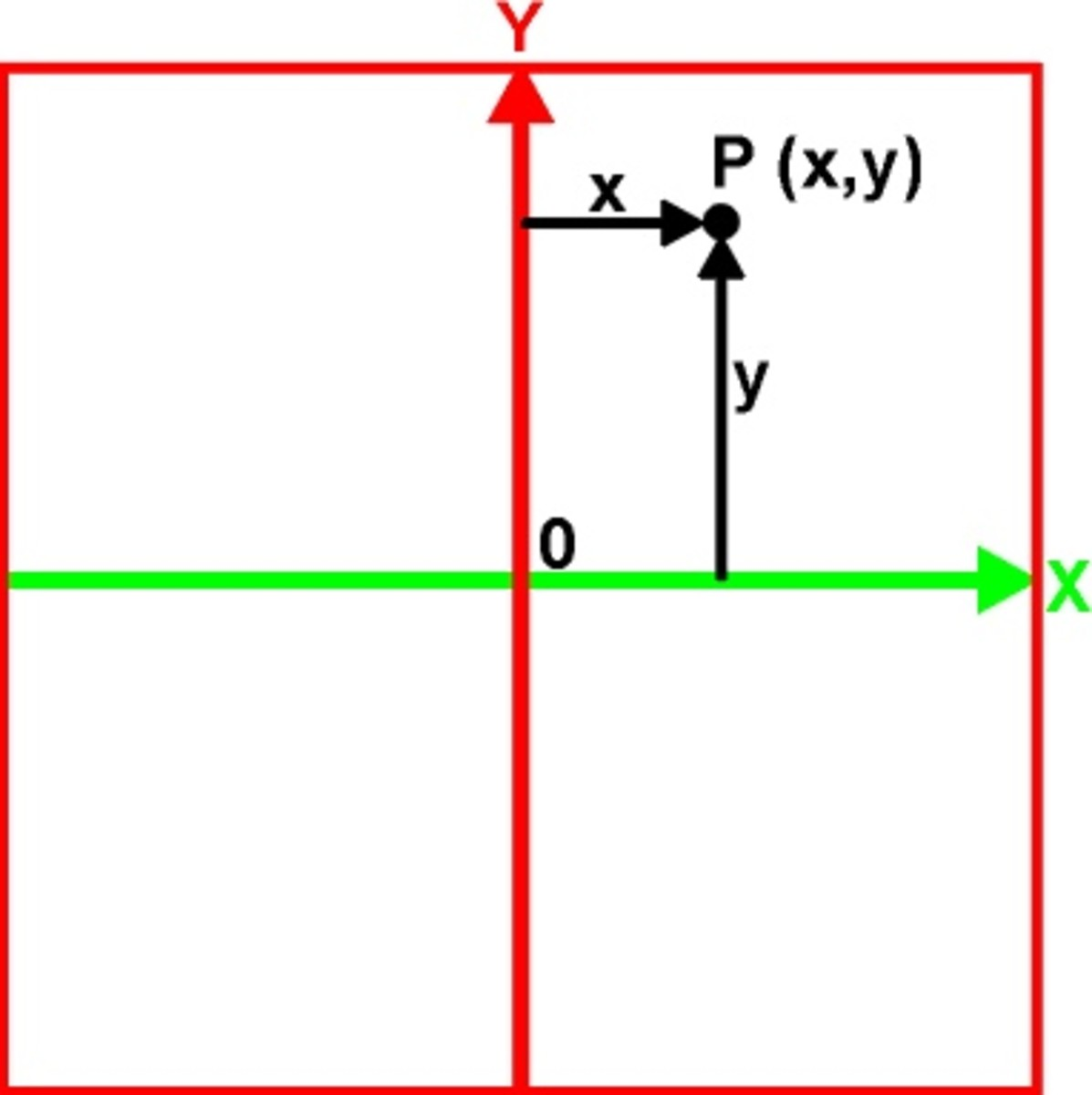Fig. 3. One plane of 2D space

Fig. 3 shows the x,y plane, indicted by a square that contains the x,y axes of 2D space. These axes are 90 degrees to each other. Any point on the plane is located by two numbers (x,y). X is the distance from the y-axis to the point. Y is the distance from the x-axis to the point. The 2D coordinate system is a single plane.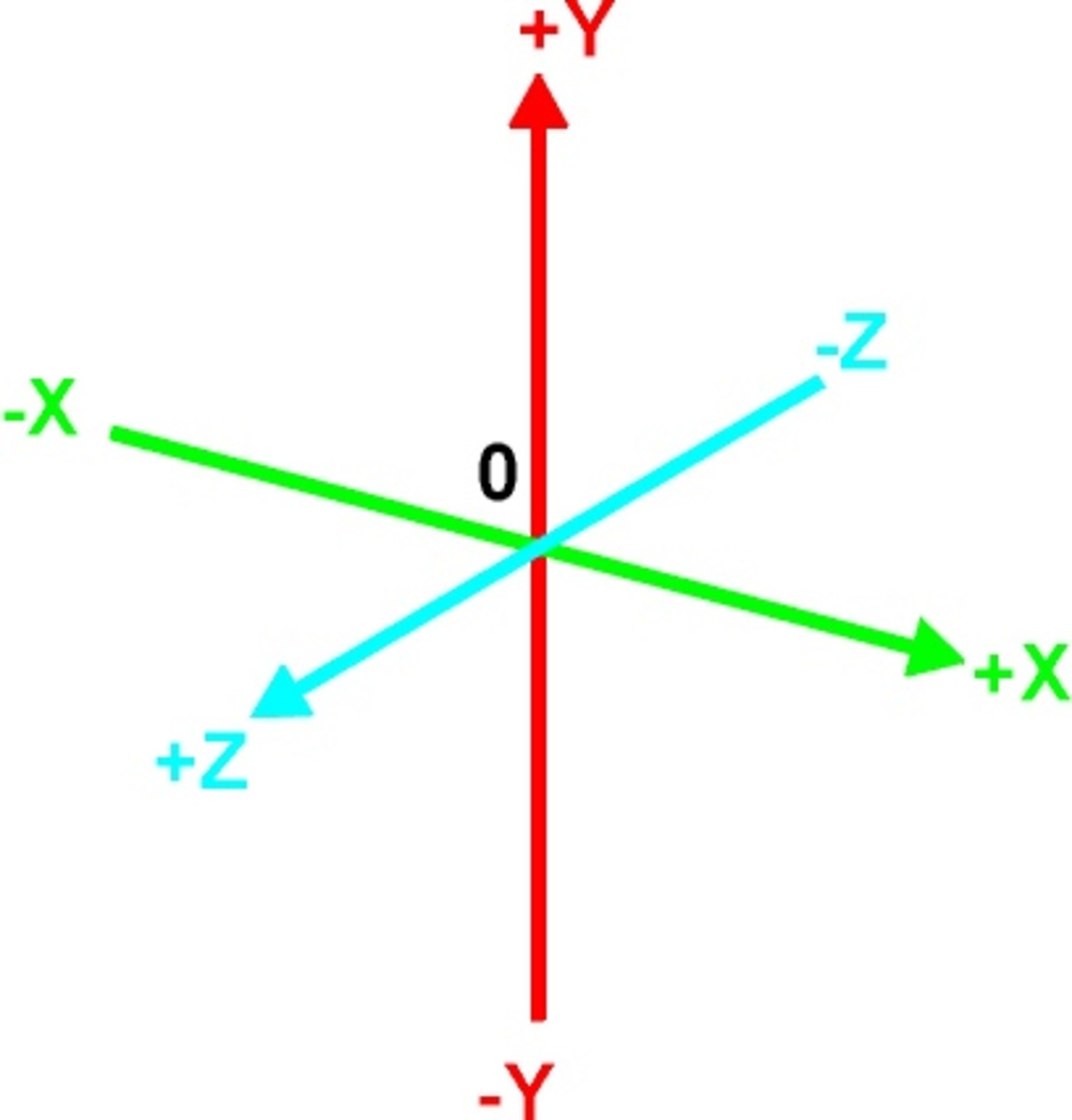Fig. 4. The X, Y, and Z axes of 3D space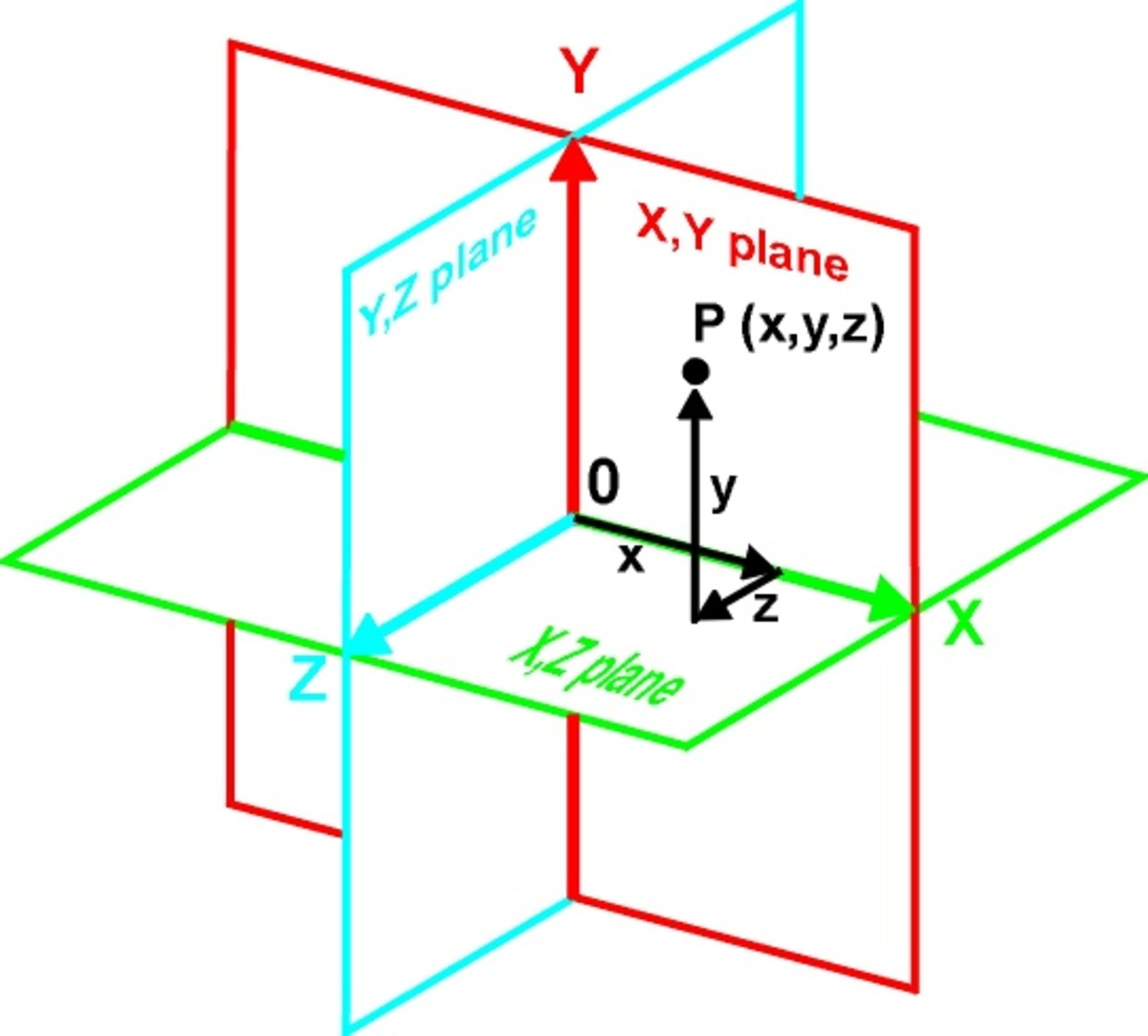Fig. 5. The three planes of 3D space

Any point in 3D space is located by three numbers (x,y,z). The 3D coordinate system consists of three planes. Here, these planes are indicated by squares and they are each 90 degrees to each other. Because we are viewing the planes at an angle and their image is flattened to the 2D surface of the page, the squares do not look like squares and the angles do not appear to be 90 degrees. However, we are used to seeing squares at an angle and can accept the drawing as representing three perpendicular squares.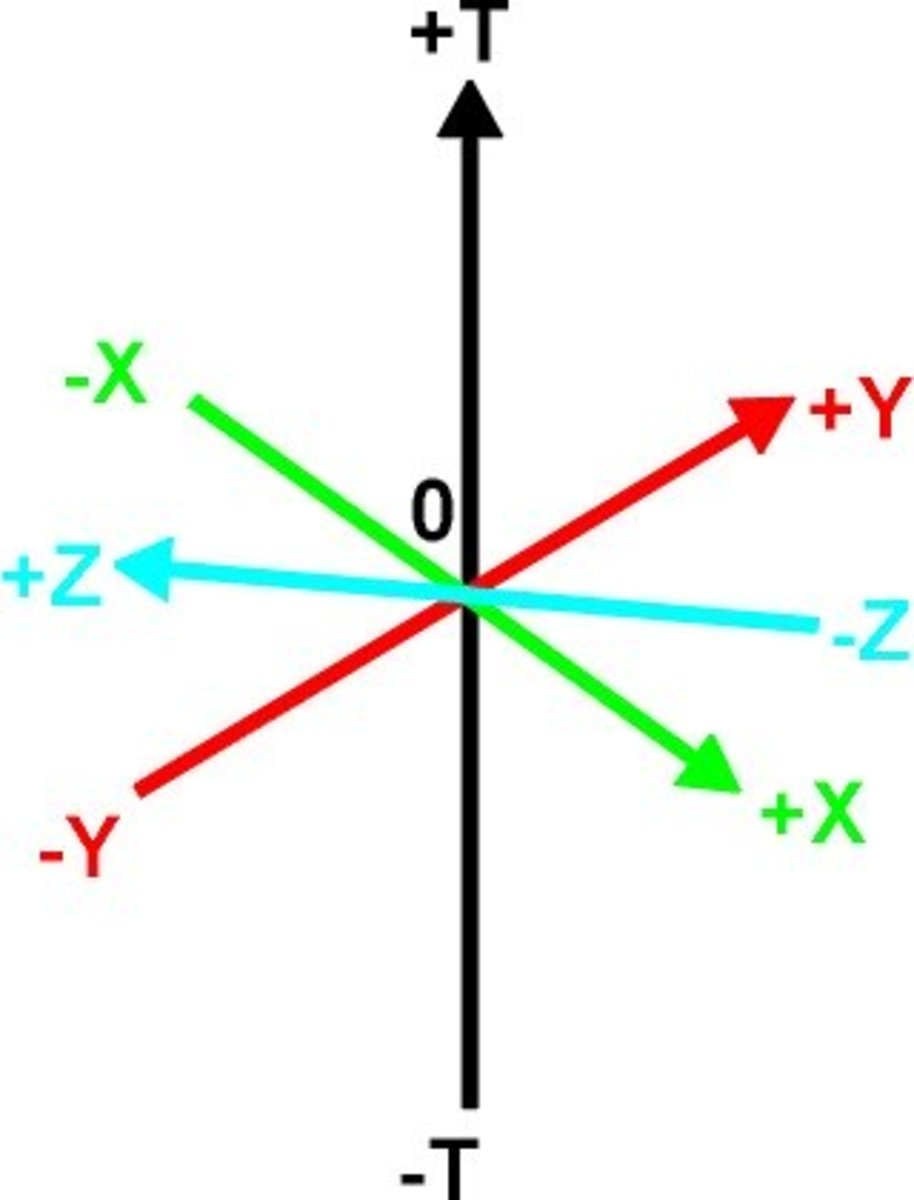Fig. 6. The four mutually perpendicular axes of 4D space. These can represent three spatial axes and one time axis or four spatial axes x,y,z,w.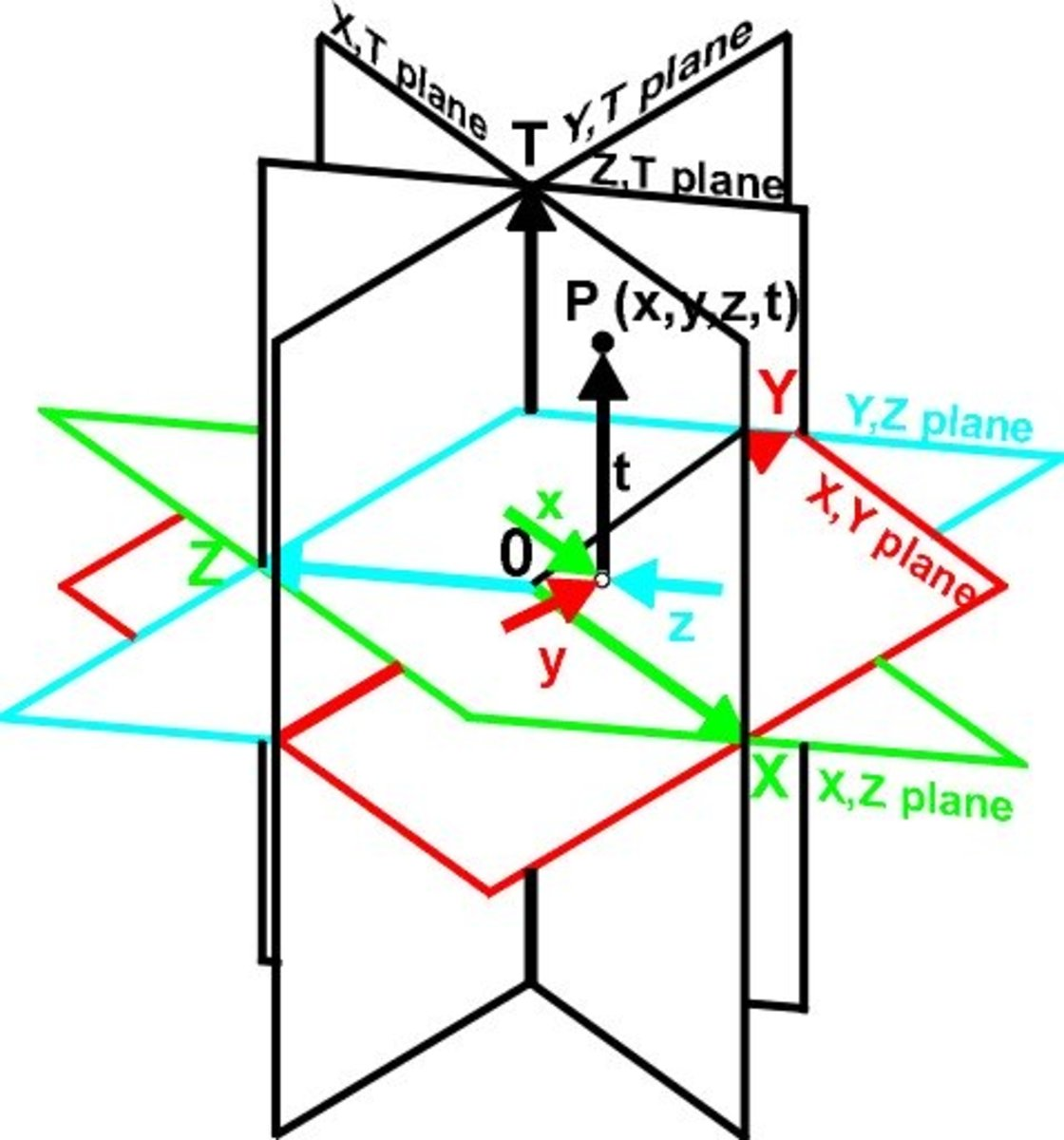Fig. 7. The three 3D planes on their 4D axes.

The 4D coordinate system consists of six planes. This equals all the paired combinations of the axes, xy, xz, xw, yw, zw and yz. That is the number of combinations of n objects taken r at a time = n!/r!(n-r)! = 4!/2!(4-2)! = 24/4 = 6.

Just as the 3D planes appear distorted when projected on a 2D surface, these planes in four dimensions are even more distorted when projected on a 2D surface. Fig. 7 shows the 2D projection of the six planes that described 4D space. Any point in 4D space is located by four numbers (x,y,z,t). A representation of 4D space is like a time-exposed photo since each 3D section occurs at a different instant in time. This -D space is the Minkowski space when the Lorentz transformations are used with this coordinate system.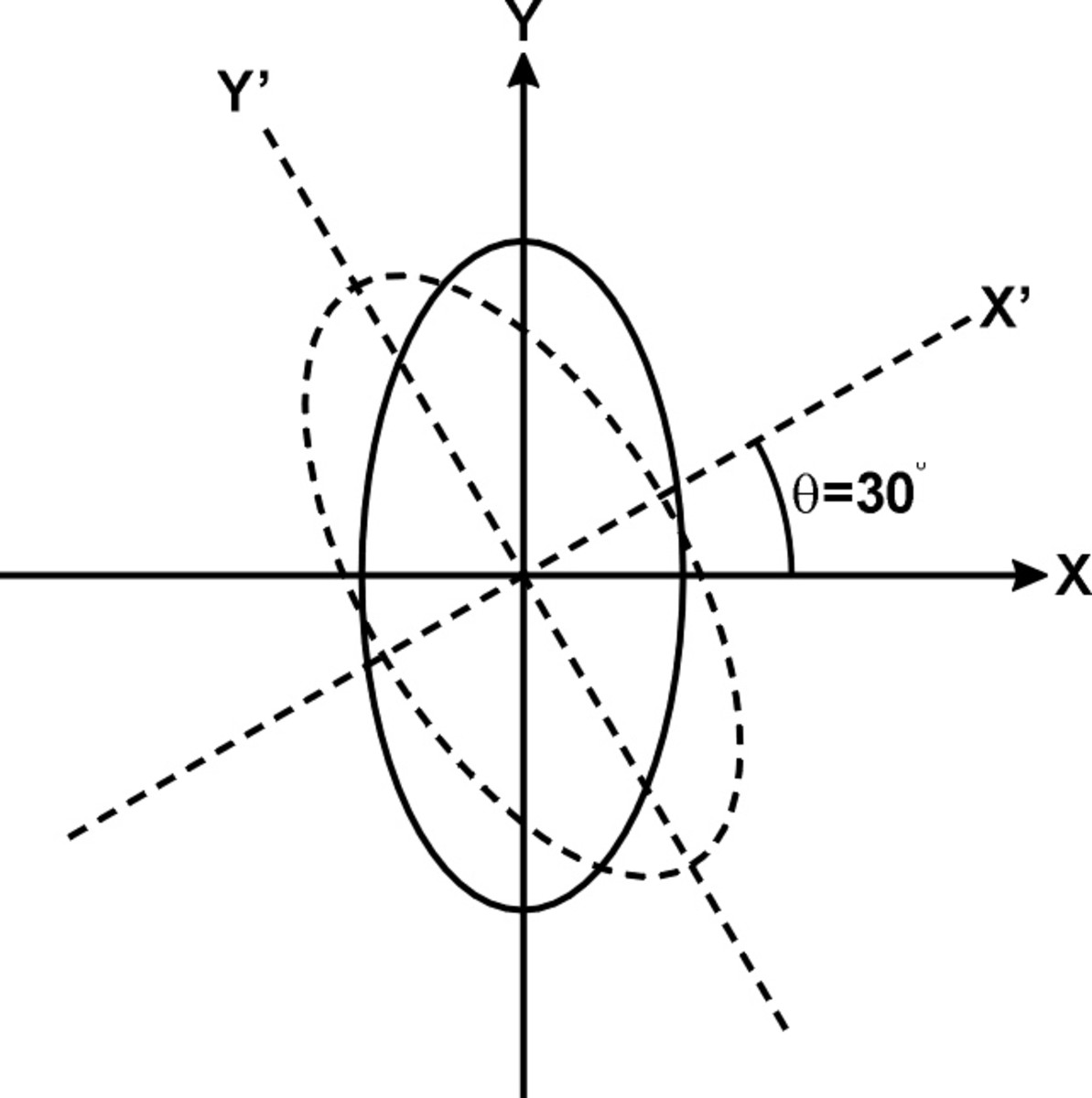Fig 8. The rotation of a 2D figure on a single plane

In analectic geometry there are two combined equations used for rotating all the 2D points in an object by the angle q, on the x,y plane. These equations are

x’ = x*cos q – y*sin q

Scroll to Continue

## Read More From Feltmagnet

and

y’ = x*sin q +y*cos q

## Using the Equations for the 3D Figure

By expanding these two equations into six equations and using points indicated by three numbers, we produce a 2D representation of a 3D object. When one plane is rotated the whole figure is rotated by the same amount. By using three different angles of rotation this representation of a three-dimensional object can be viewed from any angle.

Algorithm that produces the 3D effect:

XA=X*COS(A1)-Y*SIN(A1):

YA=X*SIN(A1)+Y*COS(A1)

XB=XA*COS(A2)-Z1*SIN(A2)

ZA=XA*SIN(A2)+Z1*COS(A2)

ZB=ZA*COS(A3)-YA*SIN(A3)

YB=ZA*SIN(A3)+YA*COS(A3)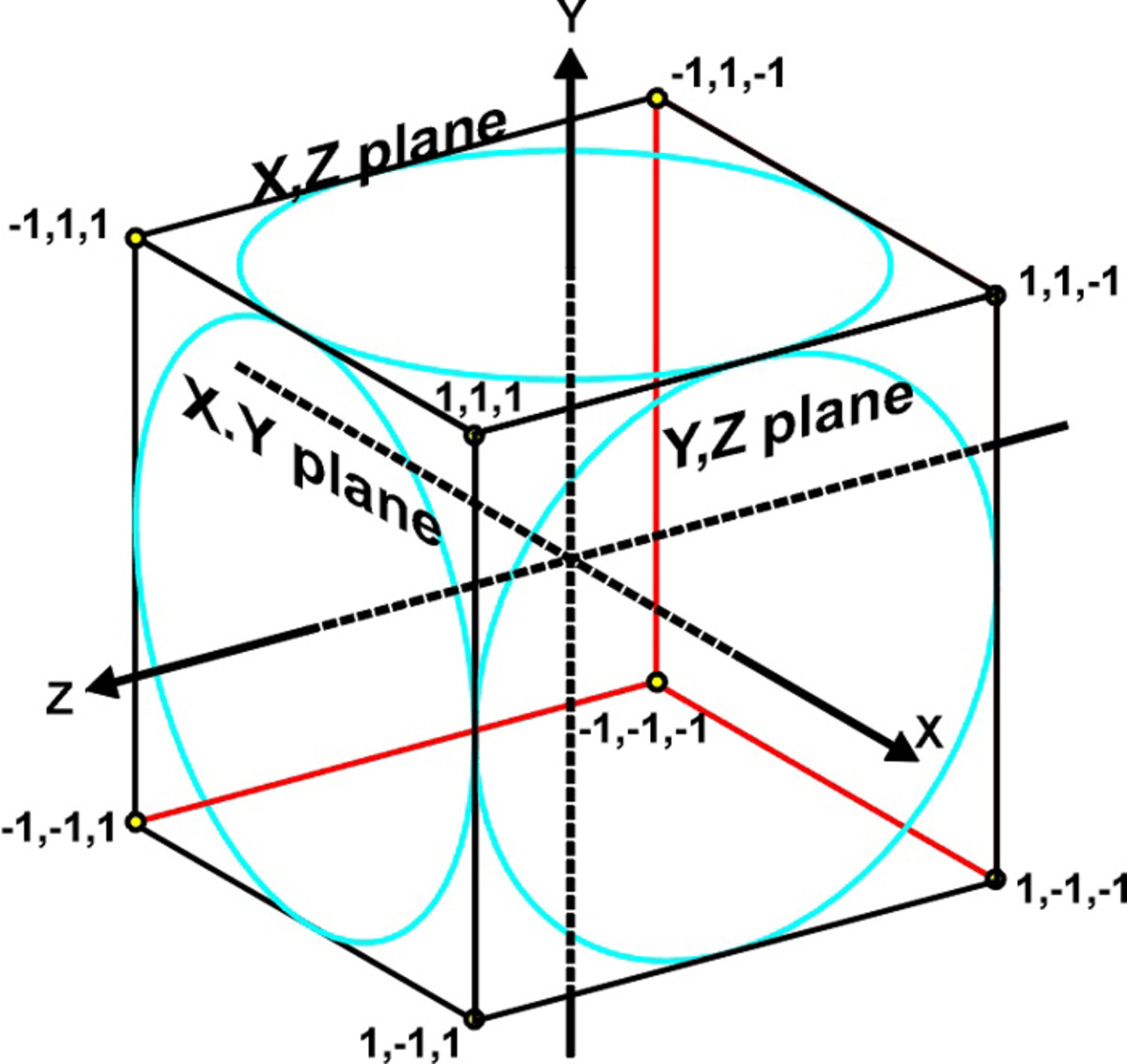Fig. 9. A cube is a 3D object.

## Using the Equations for the 4D Figure

By expanding these two equations into 12 equations and using points indicated by four numbers, we produce a 2D representation of a 4D object. By rotating any or all of the six planes of the 4D object, the representation of a four-dimensional object can be viewed from any angle.

Algorithm that produces 4D image:

ZA=Z*CQS(A1)-W*SIN(A1)

WA=Z*SIN(A1)+W*COS(A1)

YA=Y*COS(A2)-WA*SIN(A2)

WB=Y*SIN(A2)+WA*COS(A2)

XA=X*COS(A3)-ZA*SIN(A3)

ZB=X*SIN(A3)+ZA*COS(A3)

XB=XA*COS(A4)-WB*SIN(A4)

WC==XA*SIN(A4)+WB*COS(A4)

YB=YA*COS(A5)-ZB*SIN(A5)

ZC=YA*SIN(A5)+ZB*COS(A5) :

XC=XB*COS(A6)-YB*SIN(A6)

YC=XB*SIN(A6)+YB*COS(A6)

X2=K*(XC+XC*ZC/800+XC*WC/800)+158:REM adds perspective to x(k=scale)

Y2=0.8*K* (YC+YC*ZC/800+YC*WC/800)+100:REM adds perspective to y(k=scale)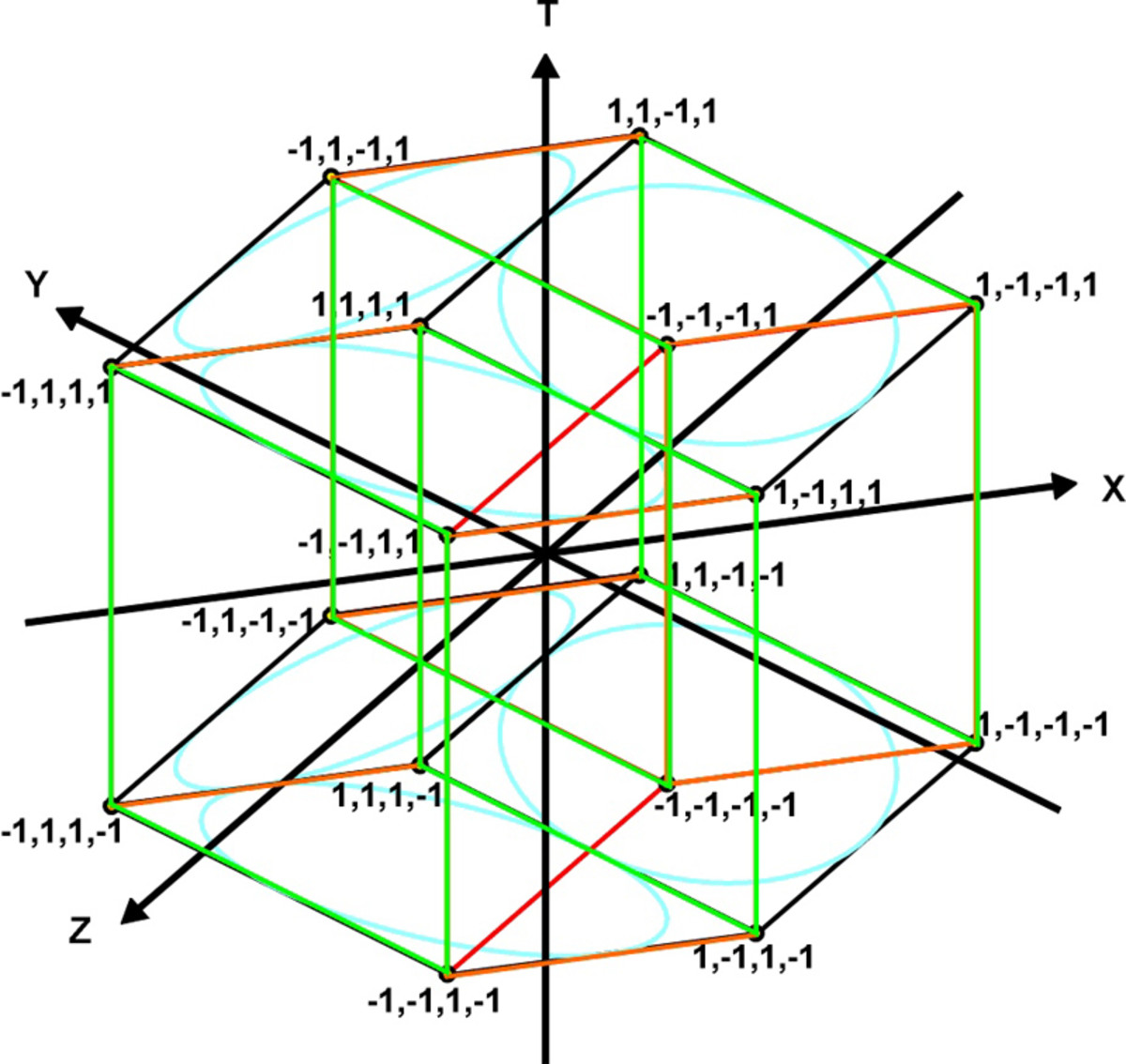Fig. 10. Tesseract or four dimensional hypercube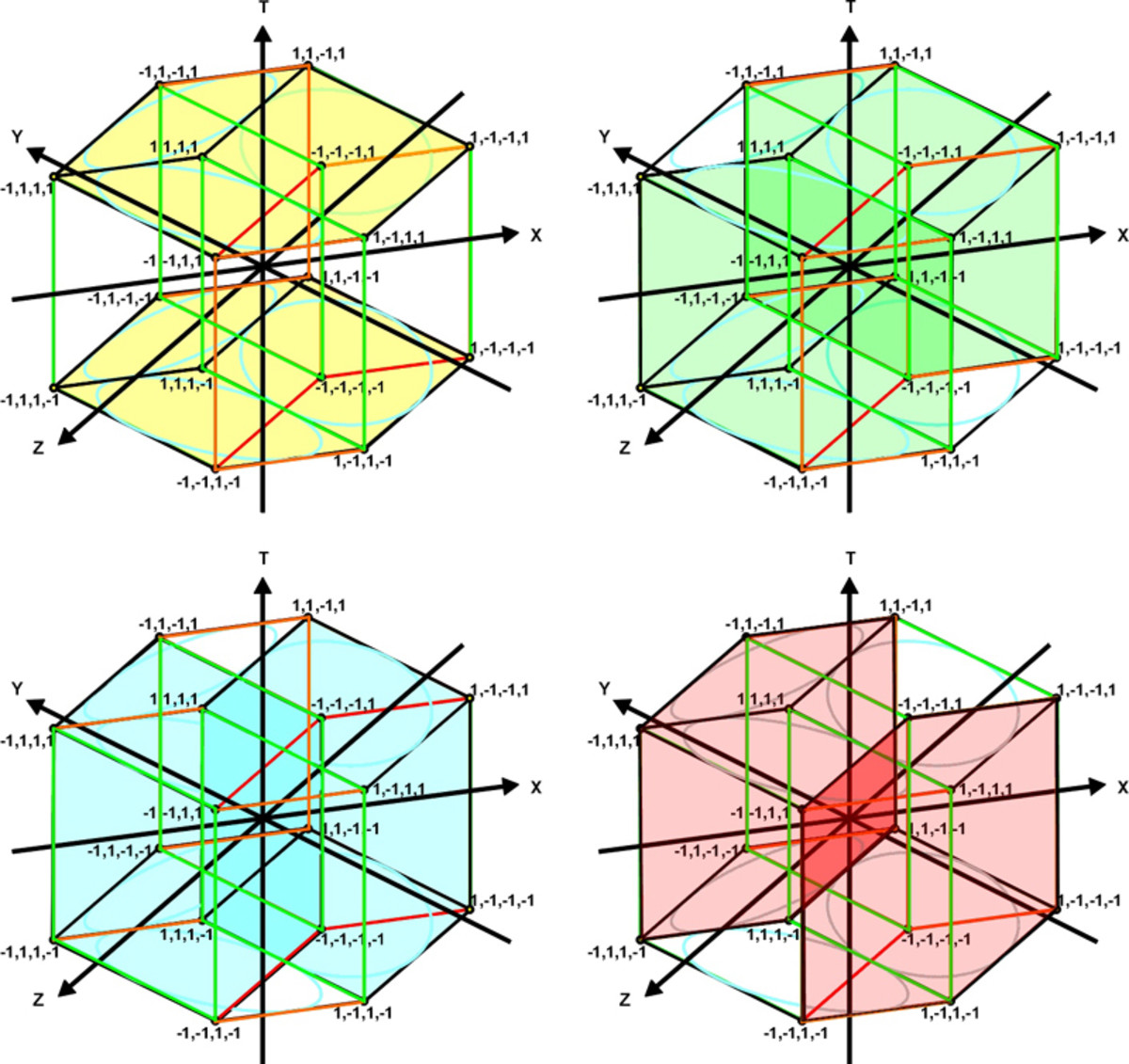Fig. 11. The hypercube is composed of eight interlaced 3D cubes.

## The Computer Program 4D-CUBE Draws the Tesseract

This program draws a two-dimensional representation of a four-dimensional hypercube. Each of the 16 points or vertices is indicated by four numbers: one number for the x-axis, the y-axis, the z-axis and the w-axis. The 32 edges are indicated by drawing a line between two vertices.

Figs. 12 and 13 show the hypercube at different degrees of rotation. Fig. 14 shows the hypercube with no rotation around any axis. Because the program has a perspective factor in it fig. 14 appears as three connected squares. Without perspective fig. 14 would appear as a single square just as a 3D cube does. In GW Basic the height of the pixels is greater than the width. When figures are viewed on the screen they are taller than they should be. By multiplying the final y by 0.8, these drawings have been adjusted so that the height and the width are proportionally corrected.

4D hypercube and the rotation of each plane

ROTATION OF ZW PLANE 10°

ROTATION OF YW PLANE 20°

ROTATION OF XZ PLANE 30°

ROTATION OF XW PLANE 40°

ROTATION OF YZ PLANE 50°

ROTATION OF XY PLANE 60°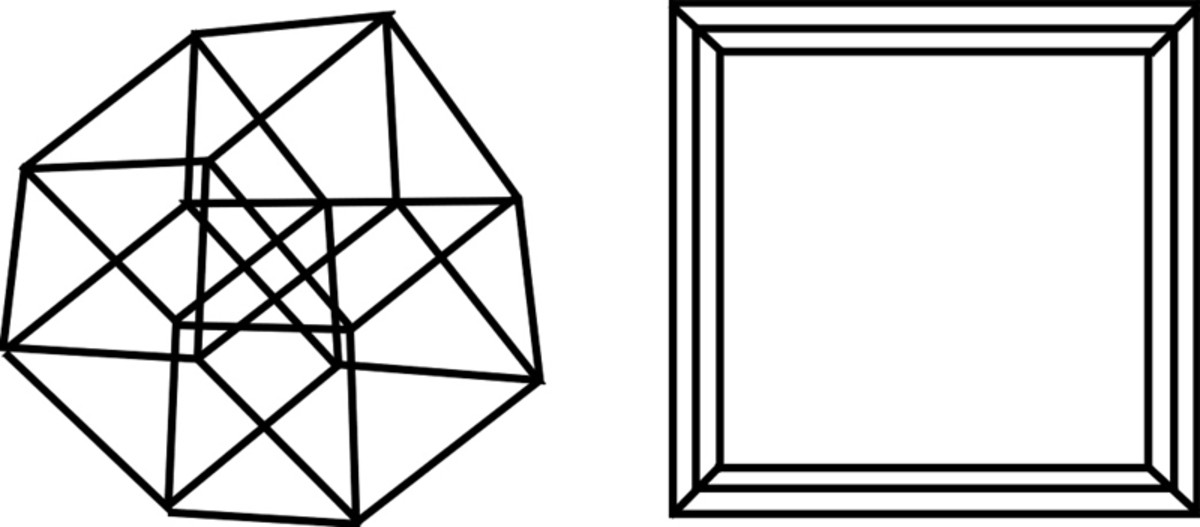Fig. 13 . . . . . . . . . . . . . . . . . . . . . . . . . . . . . .Fig 14

4D hypercube and rotation of planes . . . 4D hypercube and rotation of planes

ROTATION OF ZW PLANE 50° . . . . . . . . ROTATION OF ZW PLANE 0°

ROTATION OF YW PLANE 50° . . . . . . . ROTATION OF YW PLANE 0°

ROTATION OF XZ PLANE 50° . . . . . . . . ROTATION OF XZ PLANE 0°

ROTATION OF XW PLANE 0° . . . . . . . . ROTATION OF XW PLANE 0°

ROTATION OF YZ PLANE 0° . . . . . . . . ROTATION OF YZ PLANE 0°

ROTATION OF XY PLANE 0° . . . . . . . . ROTATION OF XY PLANE 0°

## The Computer Program 4D-CUBE in GW Basic

100 CLS:REM 4D-CUBE 110 DIM X(300)

120 DIM Y(300)

130 DIM Z(300)

140 DIM W(300)

150 INPUT "SCALE (suggest 1)";K

160 INPUT "ROTATION OF ZW PLANE IN DEGREES";Al

170 INPUT"ROTATION OF YW PLANE IN DEGREES";A2

180 INPUT "ROTATION OF XZ PLANE INDEGREES";A3

190 INPUT "ROTATION OF XW PLANE IN DEGREES";A4

200 INPUT "ROTATION OF YX PLANE IN DEGREES";A5

210 INPUT "ROTATION OF XY PLANE IN DEGREES";A6

230 A1=A1/57.29577951 converts degrees to radians

240 A2=A2/57.29577951

250 A3=A3/57.29577951

260 A4=A4/ 57,29577951

270 A5=A5/57.29577951

280 A6=A6/57.29577951

290 SCREEN 1,0: CLS:KEY OFF:COLOR 0,1 320 FOR N=I TO 2

340 IF X=1.000 THEN 530

350 ZA=Z*CQS(A1)-W*SIN(A1) :REM Algorithm that produces 4D image

360 WA=Z*SIN(A1)+W*COS(A1)

370 YA=Y*COS(A2)-WA*SIN(A2)

380 WB=Y*SIN(A2)+WA*COS(A2)

390 XA=X*COS(A3)-ZA*SIN(A3)

400 ZB=X*SIN(A3)+ZA*COS(A3)

410 XB=XA*COS(A4)-WB*SIN(A4)

420 WC==XA*SIN(A4)+WB*COS(A4)

430 YB=YA*COS(A5)-ZB*SIN(A5)

440 ZC=YA*SIN(A5)+ZB*COS(A5) :

450 XC=XB*COS(A6)-YB*SIN(A6)

460 YC=XB*SIN(A6)+YB*COS(A6)

470 if n=1 then 540

480 X2=K*(XC+XC*ZC/800+XC*WC/800)+158:REM adds perspective to x(k=scale)

490 Y2=0.8*K* (YC+YC*ZC/800+YC*WC/800)+100:REM adds perspective to y(k=scale)

500 NEXT N

505 IF W=40 THEN 1000: REM highlights one 3D cube

510 LINE (X1, Y1)-(X2,Y2),3:REM draws figure

520 GOTO 320

530 END

540 X1=K* (XC+XC*ZC/800+XC*WC/800)+158:REM adds perspective to x(k=scale)

550 Y1=0.8*K*(YC+YC*ZC/800+YC*WC/800)+100:REM adds perspective to y(k=scale)

560 GOTO 500

600 DATA -40,-40,40,-40,40,-40,40,-40

610 DATA -40, -40, 40, 40, 40, -40, 40, 40

620 DATA 40,-40,40,-40,40,40,40,-40

630 DATA 40,-40/40,40,40,40,40,40

640 DATA 40,40,40,-40,-40„40,40,-40

650 DATA 40,40,40,40,-40,40,40,40

660 DATA -40,40,40,-40,-40,-40,40,-40

670 DATA -40 ,40. 40, 40, -40, -40, 40, 40

680 DATA -40,-40,-40,-40,40,-40,-40,-40

690 DATA -40, -40, -40, 40, 40, -40, -40, 40

700 DATA 40,-40,-40,-40,40,40,-40,-40

710 DATA 40,-40,-40, 40, 40, 40,-40, 40

720 DATA 40, 40, -40, -40, -40, 40, -40, -40

730 DATA 40,40,-40,40,-40,40,-40,40

740 DATA -40,40,-40,-40,-40,-40,-40,-40

750 DATA -40,40,-40,40,-40,-40,-40,40

760 DATA -40, -40, 40, -40, -40,-40,-40,-40

770 DATA -40,-40,40,40,-40,-40,-40,40

780 DATA 40, -40, 40, -40, 40, -40, -40, -40

790 DATA 40, -40, 40, 40, 40, -40, -40, 40

800 DATA 40, 40,40,-40,40,40,-40,-40

810 DATA 40,40,40,40,40,40,-40,40

820 DATA -40,40,40,-40,-40,40,-40,-40

830 DATA -40, 40, 40,40, 40 , 40, -40, 40

840 DATA -40,-40,40,-40,-40,-40,40,40

850 DATA 40,-40,40,-40,40,-40, 40,40

860 DATA 40,40,40,-40,40,40,40,40

870 DATA -40,40,40,-40,-40,40,40,40

880 DATA -40,-40,-40,-40,-40,-40,-40,40

890 DATA 40,-40, -40, -40, 40, -40, -40, 40

900 DATA 40,40,-40,-40,40,40,-40,40

910 DATA -40,40,-40,-40,-40, 40,-40, 40

920 DATA 1000,1000,1000,1000

1000 LINE(X1,Y1)-(X2,Y2),2

1010 GOTO 320

## The Computer Program 4D-Plane

This program draws a two-dimensional representation of the six planes of the four axes. Fig. 15 shows the six planes and the coordinates in 4D space. A layout like this is helpful before drawing any 4D figure. By using six different angles of rotation, this representation of four-dimensional planes can be viewed from any angle. When all the angles are at zero we see the x.y plane as a square. All the other planes are edge-on.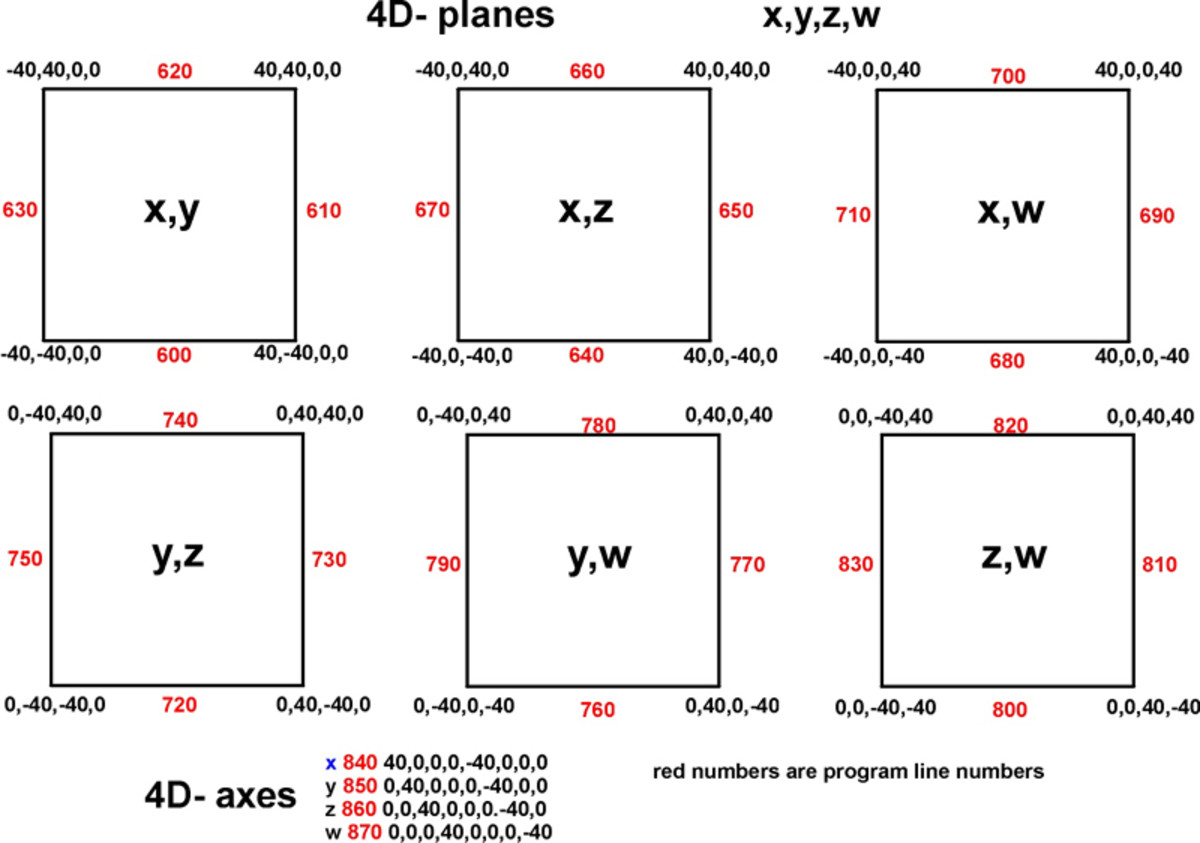Fig 15. The six planes of 4D space

Fig. 15 lays out all the points in the 4D coordinate system. These are used in the computer program 4D-PLANE

In figs. 16 to 18 we see 3D planes drawn with blue lines, while all the planes containing the w-axis are drawn in red. When we run the computer program the 3D planes are drawn with white lines while all the planes containing the w-axis are drawn in purple. In GW Basic the height of the pixels is greater than the width. When figures are viewed on the screen they are taller than they should be. By multiplying the final y by 0.8, these drawings have been adjusted so that the height and the width are proportionally corrected.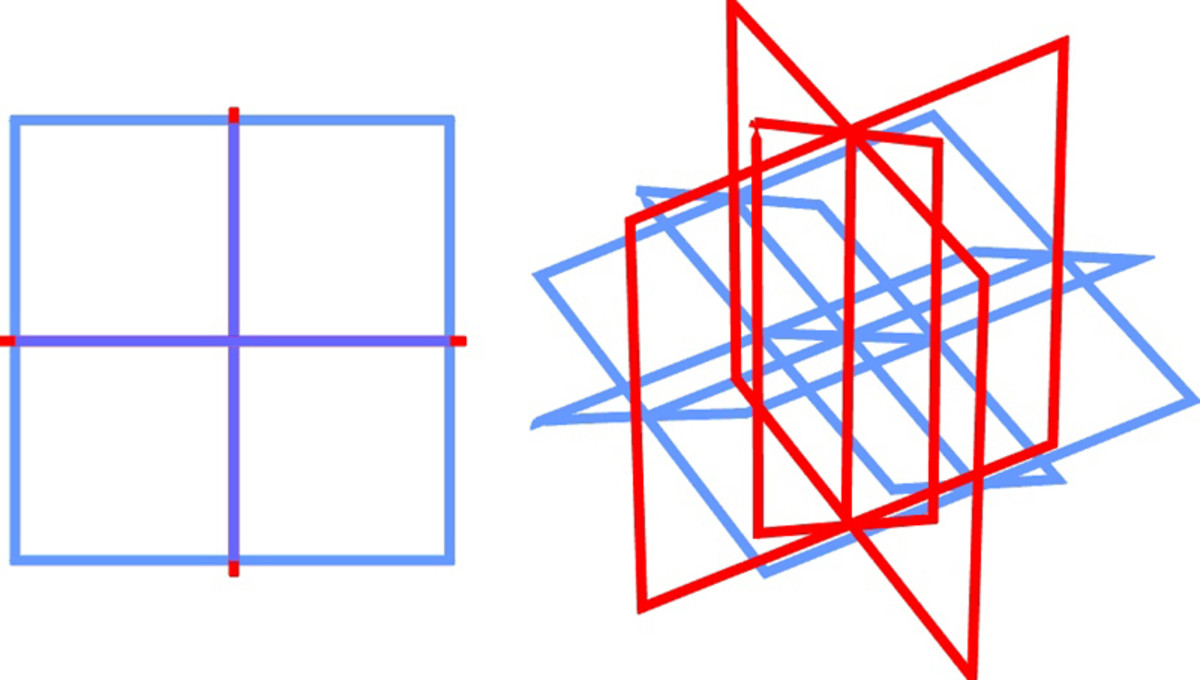Fig.16. . . . . . . . . . . . . . . . . . . . . . . . . . . . . Fig. 17

4D planes and the rotation of planes 4D planes and the rotation of planes

ROTATION OF ZW PLANE 0° . . . . . . . . ROTATION OF ZW PLANE 0°

ROTATION OF YW PLANE 0°. . . . . . . . ROTATION OF YW PLANE 0°

ROTATION OF XZ PLANE 0° . . . . . . . . ROTATION OF XZ PLANE 30°

ROTATION OF XW PLANE 0° . . . . . . . ROTATION OF XW PLANE 50°

ROTATION OF YZ PLANE 0° . . . . . . . .ROTATION OF YZ PLANE 70°

ROTATION OF XY PLANE 0° . . . . . . . . ROTATION OF XY PLANE 90°

4D coordinate planes and the rotation of each plane

ROTATION OF ZW PLANE 10°

ROTATION OF YW PLANE 20°

ROTATION OF XZ PLANE 30°

ROTATION OF XW PLANE 40°

ROTATION OF YZ PLANE 50°

ROTATION OF XY PLANE 60°

This figure is complex and hard to visualize. To help in the visualization of this figure, fig. 19 is separated into two parts. First are the 3D planes with the x,y,z axis. Second are the planes containing the w axis.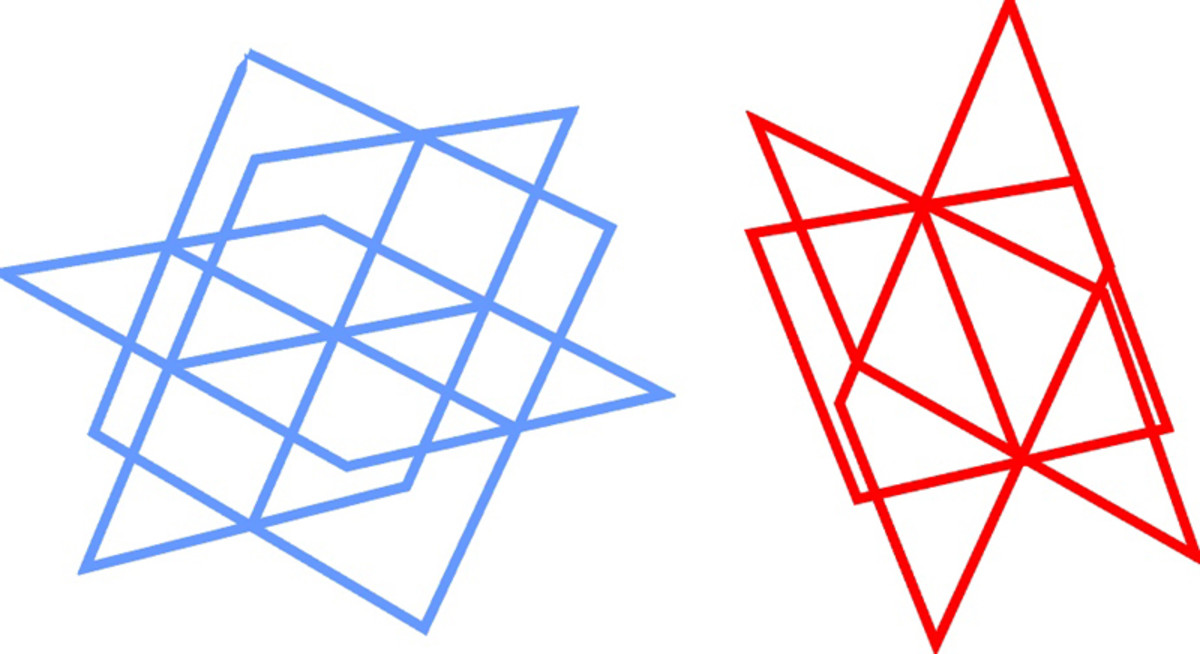Fig. 19. Two parts of a 4D coordinate system

## Computer Program 4D-PLANE

100 CLS:REM 4D-PLANE

110 DIM XC300) . 120 DIM Y(300) 130 DIM Z(300) 140 DIM W(300) 150 INPUT "SCALE";K

160 INPUT "ROTATION OF ZW PLANE IN DEGREES";Al

170 INPUT "ROTATION OF WY PLANE IN DEGREES";A2

180 INPUT "ROTATION OF XZ PLANE IN DEGREES"; A3

190 INPUT "ROTATION OF XW PLANE IN DEGREES";A4

200 INPUT "ROTATION OF YZ PLANE IN DEGREES";A5

210 INPUT "ROTATION OF XY PLANE IN DEGREES";A6

230 A1=A1/57. 2957795* :REM converts degrees to radians

240 A2=A2/57.29577951*

250 A3 =A3/57. 29577951*

260 A4=A4/57.29577951*

270 A5=A5/57,29577951*

280 A6=A6/57.29577951*

290 SCREEN 1,0:CLS:KEY OFF:COLOR 0,1

320 FOR.N=1 TO 2

340 IF X=1000 THEN 530

350 ZA=Z*COS(A1)-W#SIN(A1):REM algorithms to produce 2D representation of 4D object

360 WA=Z*SIN(A1)+W*COS(A1)

370 YA=Y*COS(A2)-WA*SIN(A2)

380 WB=Y*SIN(A2)+WA*COS(A2)

390 XA=X*COS(A3)-ZA*SIN(A3)

400 ZB=X*SIN(A3)+ZA*COS(A3)

410 XB=XA*COS(A4)-WB*SIN(A4)

420 WC=XA*SIN(A4)+WB*COS(A4)

430 YB=YA*COS(A5)-ZB*SIN(A5)

440 ZC=YA*SIN(A5)+ZB*COS<A5)

450 XC=XB*COS(A6)-YB*SIN(A6)

460 YC=XB*SIN(A6)+YB*COS(A6)

470 IF N=l THEN 540

480 X2=K*(XC+XC*ZC/800+XC*WC/800)+158

490 Y2=0.8*K*(YC+YC*ZC/800+YC*WC/800)+100

500 NEXT N

505 IF W= 40 OR W= -40 THEN 1000

510 LINE (X1,Y1)-(X2,Y2),3

520 GOTO 320

530 END

540 X1=K*(XC+XC*ZC/800+XC*WC/800)+158

550 Y1=0.8*K*(YC+YC*ZC/8OO+YC*WC/800)+100

560 GOTO 500

600 DATA - 40,- 40„0,0 , 40,- 40,0,0

610 DATA 40,- 40,0,0,40,40,0,0

620 DATA 40,40,0,0,-40,40,0,0

630 DATA - 40,40,0,0,- 40,- 40,0,0

640 DATA - 40 ,0,40, 0, 40, 0., - 40, 0

650 DATA 40, 0,- 40,0,40,0, 40,0

660 DATA - 40,0,40,0 , 40,0,40, 0

670 DATA - 40, 0, 40, 0, - 40, 0, - 40, 0

680 DATA 0, - 40, - 40, 0, 0, 40, - 40, 0

690 DATA 0,40,- 40,0,0,40,40,0

700 DATA 0,40,40,0,0, - 40, 40, 0

710 DATA 0,- 40, 40, 0,0,- 40,,- 40,0

720 DATA - 40,0,0,- 40,40,0,0,-40

730 DATA 40,0,0,- 40,40,0,0,40

740 DATA 40,0,0,40,- 40,0,0,40

750 DATA - 40,0.0,40,- 40,0,0,- 40

760 DATA 0,- 40,0,- 40,0,40,0,- 40

770 DATA 0,0, 0, - 40 , 0, 40, 0, 40

780 DATA 0,40.0, 40,.0,40,0,40

790 DATA 0, -40, 0, 40, 0, -40,, 6, - 40

800 DATA 0,0, 40, - 40, 0, 0, - 40, - 40

810 DATA 0,0,40, 40, 0., 0, 40, - 40

820 DATA 0,0, 40, 40, 0, 0, - 40, 40

830 DATA 0,0,- 4,40,0,0,- 40,- 40

840 DATA 40, 0, 0, 0, - 40, 0, 0.0

850 DATA 0,40,0,0,0,- 40,0,0

860 DATA 0,0,40,0,0,- 40,0

870 DATA 0,0,0,40,0,0,0,- 40

920 DATA 1000,1000,1000,1000

1000 LINE (X 1, Y1) – (X2, Y2) , 2

1010 GOTO 320

This program has built-in perspective so that lines more distant from the eye are smaller. To remove this perspective, change lines 480, 490, 540, and 550 to:

480 X2=K*(XC)+158

490 Y2=K*(YC)+100

540 X1=K*(XC)+158

550 Y1=K*(YC)+100

This is also true for 4D-CUBE. The perspective can be removed from that program by the same lines in it.

An approximate 4D drawing can be made by drawing a 3D drawing of an object twice and then connecting the point with lines. Fig. 20 shows the 4D coordinate system drawn this way.

Fig. 21 shows the 4D tetrahedron, cube and octahedron drawn this way.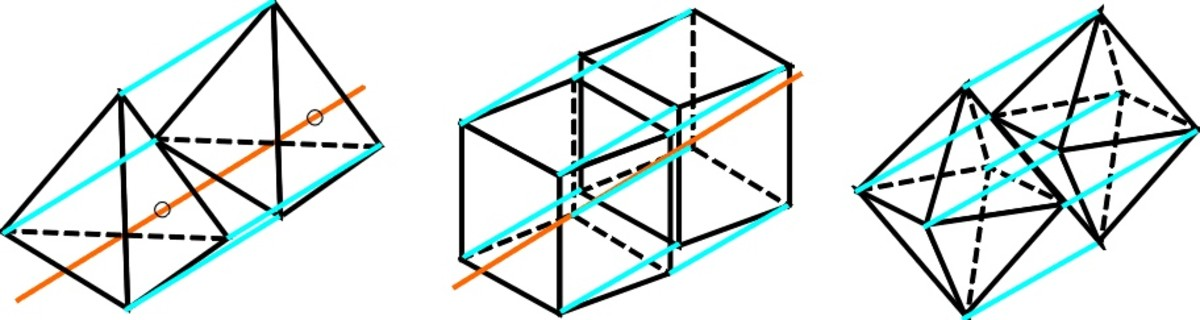Fig. 21. Four-dimensional tetrahedron, cube and octahedron

## Expand Your Understanding

By understanding these principles you can draw all sorts of 4D figures. These can be used to study and understand multiple-dimensional systems.

Mara Alexander from Los Angeles, California on February 27, 2015:

So kewl, this is absolutely awesome. Thank you for sharing

I voted it up

Bennimus - Continuing on October 29, 2014:

I also forgot to mention. The connect-the-dots trick will work on cubes, but you can't use it on tetrahedrons. You will get a "tetrahedronal prism". A proper 5-cell has 5 vertices. Same for octohedrons. It WILL NOT WORK unless you want to end up with a prism.

Bennimus on October 29, 2014:

About the 4D graph. There's a bit of a pattern in 2D representations of higher-dimensional graphs.

Generally, the Z axis goes up. In 2D drawings, Y goes up. So where does Y go? It gets squished down a little bit with the X axis.

The same thing happens when we introduce what's properly known as a W axis. When the W axis enters the scenario, It points up, and it squishes the Z axis alongside the Y and X axes.

All in all it really doesn't matter so long as you have 4 axes, but if you're ever going to do measurements of hypercubes, this is the easiest way to do it.

Rahul on August 01, 2013:

Its really so good, but i know this, can you tell me something about 4-D sphere. My name is Rahul and my age is 14. If you can tell me something about 4-D sphere than please tell me, this is my ID- planetchachi@gmail.com

Thank You Sir

Casper on December 30, 2012:

Excellent!, however, a 4d tetrahedron has only 5 corners!

In your example of a 4d tetrahedron you have made 2 normal tetrahedrons connected to each other bij 90 degree angles into the fourth dimension.

Zack on February 29, 2012:

I could not do any of that! I'm only in 7th grade, and love math, and geometric shapes, but that made no sense. Could you make that clearer, please? my email is lemanski_z@yahoo.com. Thx, Zack.

sve on February 20, 2012:

You made it so simple, it is genius. This is the best explanation of a Fundamental Principle I have seen. Thanks. Keep going.

p johnny joe on November 22, 2011:

sir,it was awesome to see four dimensional figure made by you,when it is going to come in to action,can we make things useful to humanbeings by these figures,100% of the things are made up of 3dimensional,iam a mathematics teacher iam curious to know about it please write in detail to my id johnnyjoe2006@yahoo.com thank you

toxiKrystal on June 06, 2010:

very clear and concise. suprising, considering the amount of usefull informatin here, as well as the complex nature of fourth-dimentional space itself.

i acquired useful knowledge and i am sure there is more to learn here. Bookmarked for sure ^-^

... aren't 4dimentional objects stunningly beautiful ^-^ you definitely aided my task of drawing them by hand.

much thanks

-krys

Mood on May 12, 2010:

Sir you are awesome and passionate, really benefited from this article, thank you and keep up the awesome work coming !